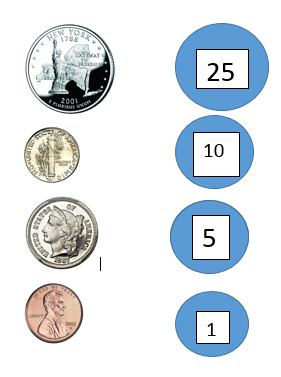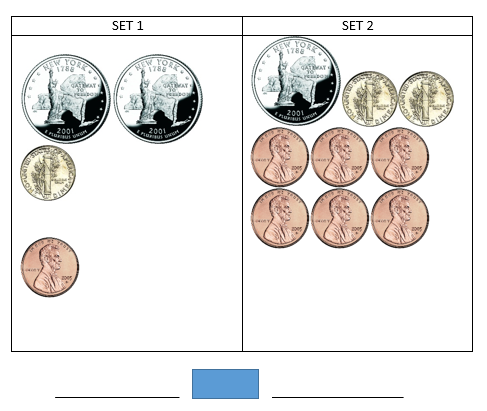# Comparing Two Sets of Money

Instructor: Jaimee Arnold

Jaimee has worked in elementary school and has her Master's +30.

In this lesson, you will be comparing two sets of money. You'll learn how to use circles and scrap paper to find the amount of money in each set, as well as everyday math symbols to write an equation for the money sets.

## Sets of Money

The money we'll use in this lesson will focus on \$1 bills and coins, including the quarter, dime, nickel, and penny. It is important to remember what each coin is worth so you can add up the total amount of money in each set. A helpful hint when adding money is to use a piece of paper and write the amount a coin is worth inside a circle. Use this illustration as an example of what your work could look like.## Math Vocabulary and Symbols

We'll use the same math vocabulary to compare money as we do to compare numbers. Using the correct symbol with the correct terms can be a little tricky.

Greater than (>): When using this symbol, we write the larger number first, then the greater than symbol (>), then the smaller number. For example, 5 > 3 means 5 is greater than 3.

Less Than (<): When using this symbol, we write the smaller number first, then the less than symbol (<), than the larger number. For example, 10 < 15 means 10 is less than 15.

Equal (=): This symbol means that two numbers are equal to each other, like 13 = 13.

## Adding and Comparing Money

There are two steps you must follow when completing money problems. First, you need to determine the total amount of money in each set. Take your time and double check your work. Second, you need to compare the two totals.

When comparing the totals, look at the whole dollars first. If your total for one set is \$1.25 and the total for the other set is \$3.50, you do not need to look at the cents side because \$3 is worth more than \$1. But if your dollar amounts are the same, then you need to look at the cents.

Let's try an example. Take a few minutes to look at the picture below.First, add the total amount of money in each set. This would be a good time to pull out some scrap paper and write the amount each coin is worth in a circle. Then, show your addition work. Remember to put the 1-digit numbers in the 'ones' column and the 2-digit numbers in the 'tens and ones' column.

To unlock this lesson you must be a Study.com Member.

### Register to view this lesson

Are you a student or a teacher?

### Unlock Your Education

#### See for yourself why 30 million people use Study.com

##### Become a Study.com member and start learning now.
Back
What teachers are saying about Study.com

### Earning College Credit

Did you know… We have over 160 college courses that prepare you to earn credit by exam that is accepted by over 1,500 colleges and universities. You can test out of the first two years of college and save thousands off your degree. Anyone can earn credit-by-exam regardless of age or education level.